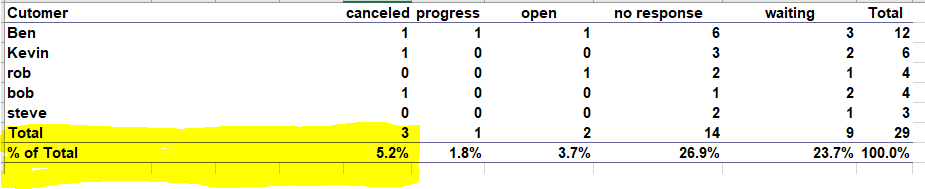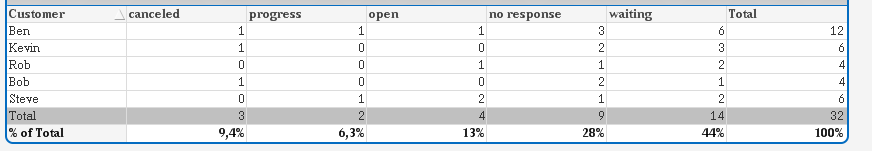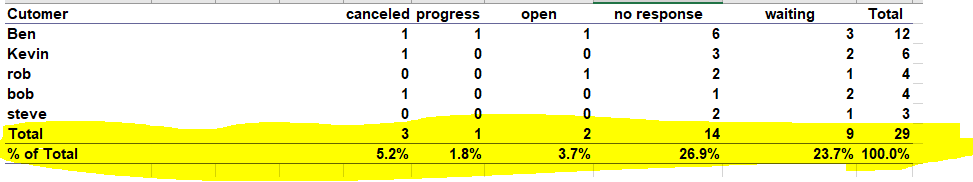New to QlikView

Discussion board where members can get started with QlikView.

Announcements
QlikWorld 2020: Join us May 11 - 14, 2020 in Phoenix, AZ. Register early and save \$400. Learn More
Highlighted
Contributor III

Totals and % totals in Qlikview straight table

Hi all,

Is there any way around to show totals and % of totals in straight table as shown in below imageCan any one help.

Thanks,

Ganesh

1 Solution

Accepted Solutions
Honored Contributor II

Re: Totals and % totals in Qlikview straight table

Ok, here the adapted solution:

LOAD * INLINE [

Dim

1

2

];

LOAD * INLINE [

Customer, Progress, Open, No Response, Waiting, Canceled

Ben, 1, 1, 6, 3, 1

Kevin, 0, 0, 3, 2, 1

Rob, 0, 1, 2, 1, 0

Bob, 0, 0, 1, 2, 1

Steve, 1, 2, 2, 1, 0

Ok];

Dimension:

=Pick(Dim,Customer,'Total')

Expression 1 for canceled:

if(Dimensionality()=0,

num(sum(Canceled)/(sum(Canceled)+sum(Progress)+sum(Open)+sum([No Response])+sum(Waiting)),'##%'),

sum(Canceled))

Expression 2 for progress:

if(Dimensionality()=0,

num(sum(Progress)/(sum(Canceled)+sum(Progress)+sum(Open)+sum([No Response])+sum(Waiting)),'##%'),

sum(Progress))

Expression 3 for open:

if(Dimensionality()=0,

num(sum(Open)/(sum(Canceled)+sum(Progress)+sum(Open)+sum([No Response])+sum(Waiting)),'##%'),

sum(Open))

Expression 4 for no response:

if(Dimensionality()=0,

num(sum(Waiting)/(sum(Canceled)+sum(Progress)+sum(Open)+sum([No Response])+sum(Waiting)),'##%'),

sum(Waiting))

Expression 5 for waiting:

if(Dimensionality()=0,

num(sum([No Response])/(sum(Canceled)+sum(Progress)+sum(Open)+sum([No Response])+sum(Waiting)),'##%'),

sum([No Response]))

Expression 6 for TOTAL:

if(Dimensionality()=0,

num(Column(1)+Column(2)+Column(3)+Column(4)+Column(5),'###%'),

Column(1)+Column(2)+Column(3)+Column(4)+Column(5))

And this is my Output:hope this helps!

12 Replies
Honored Contributor II

Re: Totals and % totals in Qlikview straight table

see attached file,

hope this helps

Contributor III

Re: Totals and % totals in Qlikview straight table

Hi Frank,

I can not open the qvw files, could you please share any useful community links.

Thanks,

Honored Contributor II

Re: Totals and % totals in Qlikview straight table

Skript:

LOAD * INLINE [

Dim

1

2

];

LOAD * INLINE [

Customer, Progress, Open, No Response, Waiting, Canceled

Ben, 1, 1, 6, 3, 1

Kevin, 0, 0, 3, 2, 1

Rob, 0, 1, 2, 1, 0

Bob, 0, 0, 1, 2, 1

Steve, 1, 2, 2, 1, 0

];

As dimension in your straight table use:

=Pick(Dim,Customer,'% of Total')

Expressions:

if(Pick(Dim,Customer,'% of Total')='% of Total',

num(sum(Canceled)/(sum(Canceled)+sum(Progress)+sum(Open)+sum([No Response])+sum(Waiting)),'##%'),

sum(Canceled))

if(Pick(Dim,Customer,'% of Total')='% of Total',

num(sum(Progress)/(sum(Canceled)+sum(Progress)+sum(Open)+sum([No Response])+sum(Waiting)),'##%'),

sum(Progress))

if(Pick(Dim,Customer,'% of Total')='% of Total',

num(sum(Open)/(sum(Canceled)+sum(Progress)+sum(Open)+sum([No Response])+sum(Waiting)),'##%'),

sum(Open))

if(Pick(Dim,Customer,'% of Total')='% of Total',

num(sum(Waiting)/(sum(Canceled)+sum(Progress)+sum(Open)+sum([No Response])+sum(Waiting)),'##%'),

sum(Waiting))

if(Pick(Dim,Customer,'% of Total')='% of Total',

num(sum([No Response])/(sum(Canceled)+sum(Progress)+sum(Open)+sum([No Response])+sum(Waiting)),'##%'),

sum([No Response]))

if(Pick(Dim,Customer,'% of Total')='% of Total',

num(Column(1)+Column(2)+Column(3)+Column(4)+Column(5),'###%'),

Column(1)+Column(2)+Column(3)+Column(4)+Column(5))

hope this helps

Honored Contributor II

Re: Totals and % totals in Qlikview straight table

and for sorting use this expression:

only({1}Dim)  --> Ascending

Honored Contributor II

Re: Totals and % totals in Qlikview straight table

Do you have any further questions?

Re: How to mark Helpful or correct reply?

Contributor III

Re: Totals and % totals in Qlikview straight table

Thanks for the valuable reply, but total count row is missing form your example how to get that?

and also % symbol is missing form my % of total rows. FYI I am using 'dimensionality()=0' instead Pick(Dim,Customer,'% of Total')='% of Total',

Thanks,

Ganesh

Contributor III

Re: Totals and % totals in Qlikview straight table

I have above mentioned question on

'total count row is missing form your example how to get that?'

sure if i am clear, will close the thread.

Thanks,

Ganesh

Honored Contributor II

Re: Totals and % totals in Qlikview straight table

Ok, here the adapted solution:

LOAD * INLINE [

Dim

1

2

];

LOAD * INLINE [

Customer, Progress, Open, No Response, Waiting, Canceled

Ben, 1, 1, 6, 3, 1

Kevin, 0, 0, 3, 2, 1

Rob, 0, 1, 2, 1, 0

Bob, 0, 0, 1, 2, 1

Steve, 1, 2, 2, 1, 0

Ok];

Dimension:

=Pick(Dim,Customer,'Total')

Expression 1 for canceled:

if(Dimensionality()=0,

num(sum(Canceled)/(sum(Canceled)+sum(Progress)+sum(Open)+sum([No Response])+sum(Waiting)),'##%'),

sum(Canceled))

Expression 2 for progress:

if(Dimensionality()=0,

num(sum(Progress)/(sum(Canceled)+sum(Progress)+sum(Open)+sum([No Response])+sum(Waiting)),'##%'),

sum(Progress))

Expression 3 for open:

if(Dimensionality()=0,

num(sum(Open)/(sum(Canceled)+sum(Progress)+sum(Open)+sum([No Response])+sum(Waiting)),'##%'),

sum(Open))

Expression 4 for no response:

if(Dimensionality()=0,

num(sum(Waiting)/(sum(Canceled)+sum(Progress)+sum(Open)+sum([No Response])+sum(Waiting)),'##%'),

sum(Waiting))

Expression 5 for waiting:

if(Dimensionality()=0,

num(sum([No Response])/(sum(Canceled)+sum(Progress)+sum(Open)+sum([No Response])+sum(Waiting)),'##%'),

sum([No Response]))

Expression 6 for TOTAL:

if(Dimensionality()=0,

num(Column(1)+Column(2)+Column(3)+Column(4)+Column(5),'###%'),

Column(1)+Column(2)+Column(3)+Column(4)+Column(5))

And this is my Output:hope this helps!

Contributor III

Re: Totals and % totals in Qlikview straight table

If i use new expressions, i am getting out put like this.But i  need output like this, I need both the rows Total and '% of Total'Thanks,

Ganesh